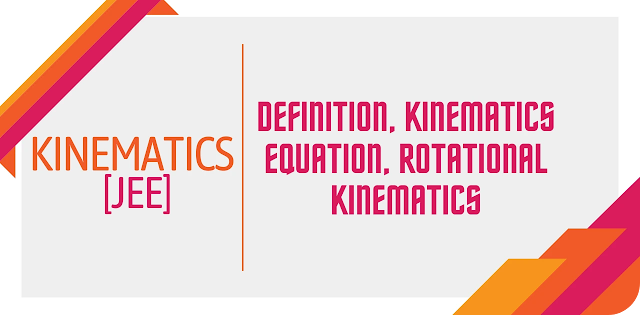# Kinematics

We can find objects in motion all around us. Even when the person is resting, the heart pumps blood through the veins. There is the motion of atoms and molecules in all the objects. There is motion when the ball is hit by a player with his bat. The branch of classical mechanics that deals with the study of the motion of the points, objects and a group of objects without considering the causes of motion are called Kinematics. The word kinematics came from the Greek word “kinesis”, meaning motion. Kinematics has its application in astrophysics to study the motion of celestial objects. It is also used in robotics, biomechanics to explain the motion of objects with joint parts such as engines, human skeletons, robotic arms and much more.Kinematics – Definition, Kinematics Equation, Rotational Kinematics

In kinematics, we study the trajectories of the objects, as well as their differential properties like velocity and acceleration.

## Reference Frames

The position of the object relative to the reference frame has to be described in order to understand the motion of the object. Mathematically, the variable ‘x’ is used to represent the position of the object. The position variable x can be described by making two choices. We can decide where x = 0 has to be put and which direction has to be taken as the positive direction. This is known as choosing the frame of reference or the coordinate system. Therefore, choosing the coordinate system or the set of axes within which the position, orientation and other properties of the object are being measured is called the frame of reference.

## Displacement

The change in the position of the object with respect to the frame of reference is called displacement. For example, if a person walks from his house to the market, the displacement is the relative distance of the market from his house (frame of reference).

## Velocity and Acceleration

The velocity of the object is defined as the displacement by the time taken. It is a vector quantity, and it has both magnitude and direction. The rate of change of velocity is called acceleration.

### Motion Graph

There are three types of motion graphs that are studied in kinematics.

1. Displacement – time graph

2. Velocity – time graph

3. Acceleration – time graph

## Motion Diagram

The pictorial representation of the motion of the object is called the motion diagram. In the same diagram, various positions of the object at equally spaced intervals are represented in a motion diagram. From the diagram we can see if the object has accelerated, retarded or at rest. We can understand that the object is getting accelerated if there is an increase in the space between the objects as time passes, and the object is getting retarded if the space between the object decreases with time.

## Kinematic Equations

There are four kinematic equations when the initial starting point is taken as the origin, and the acceleration of the object is constant.

1. v = v0 + at

2. d = (½) (v0 + v)t

3. d = v0t + (at2/2)

4. v2 = v02 + 2ad

v is the final velocity

v0 is the initial velocity

a is the constant acceleration

t is the time interval

d is the displacement

Each of the above equations has only four of the five variables. If we know the value of three variables in an equation, the fourth variable can be determined.

## Rotational Kinematics Equations

In the translational motion, we saw there are five important variables. Each of these variables will have a corresponding variable in rotational motion. The position variable x is replaced by the angle θ in a rotational motion. The initial and the final velocity is given by the angular velocity(ω), and it is measured in radians per second. The acceleration is replaced by the angular acceleration (α), which describes the rate of change of angular velocity with respect to time. Angular acceleration is measured in radians per second square. The time is represented as t even in rotational motion. The rotational kinematics equations are

1. ω = ω0 + αt

2. θ = θ0 + (½) (ω0 + ω)t

3. θ = θ0 + ω0t + (αt2/2)

4. ω 2 = ω02 + 2α (θ – θ0)

## Frequently Asked Questions on Kinematics

### What do you understand by Kinematics?

Kinematics deals with the study of the mechanical motion of the bodies without considering the forces that cause this motion.

### What is a one-dimensional motion of a body?

The motion of a body is one dimensional if it moves along a straight line.

### Define two-dimensional motion.

The motion of a body in a plane is called two-dimensional motion.

### Define three-dimensional motion.

If the body moves in a three-dimensional space, it is called three-dimensional motion.

•I bring to you the BEST for students of Class 9th - 12th. I (Balkishan Agrawal) aim at providing complete preparation for CBSE Board Exams (Maths) along with several other competitive examinations like NTSE, NSO, NSEJS, PRMO, etc. & Maths Reasoning, Vedic Maths at the school level. Wishing you great learning in all aspects of your life!

error: Content is protected !!